# SAT II Math I : Graphing Other Functions

## Example Questions

### Example Question #131 : Functions And Graphs

Simplify the following expression: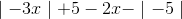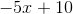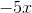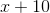Explanation:To simplify, we must first simplify the absolute values.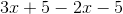Now, combine like terms:### Example Question #1 : Graphing Polynomial Functions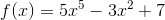Where doescross theaxis?

3

5

7

-3

-7

7

Explanation:crosses theaxis whenequals 0. So, substitute in 0 for: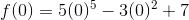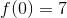### Example Question #16 : Graphing Polynomial Functions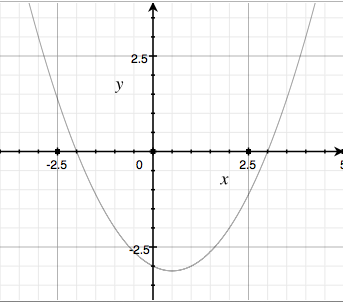Which of the following is an equation for the above parabola?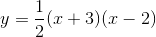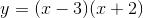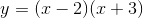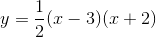Explanation:

The zeros of the parabola are atand, so when placed into the formula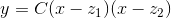each of their signs is reversed to end up with the correct sign in the answer. The coefficient can be found by plugging in any easily-identifiable, non-zero point to the above formula. For example, we can plug in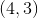which gives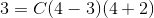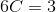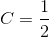### Example Question #3 : Graphing Polynomial Functions

Which equation best represents the following graph?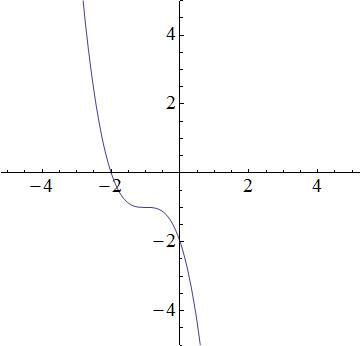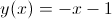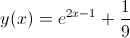None of these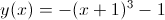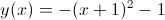Explanation:

We have the following answer choices.

1.2.3.4.The first equation is a cubic function, which produces a function similar to the graph. The second equation is quadratic and thus, a parabola. The graph does not look like a prabola, so the 2nd equation will be incorrect. The third equation describes a line, but the graph is not linear; the third equation is incorrect. The fourth equation is incorrect because it is an exponential, and the graph is not an exponential. So that leaves the first equation as the best possible choice.

### Example Question #4 : Graphing Polynomial Functions

Which of the graphs best represents the following function?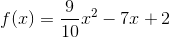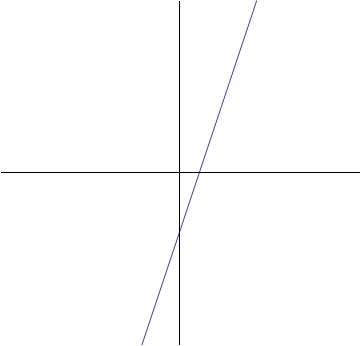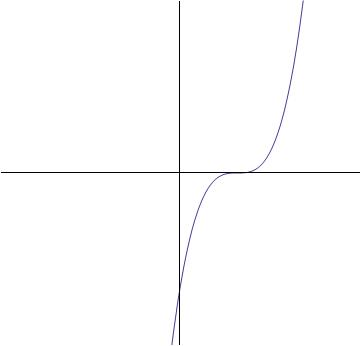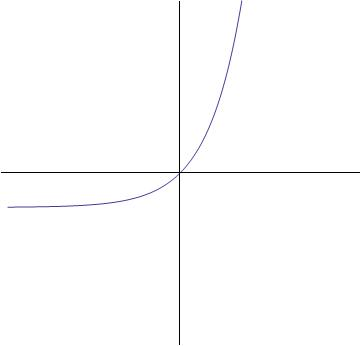None of these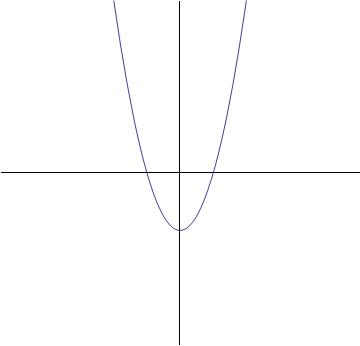Explanation:The highest exponent of the variable term is two (). This tells that this function is quadratic, meaning that it is a parabola.

The graph below will be the answer, as it shows a parabolic curve.### Example Question #7 : Graphing Polynomial Functions

Which of the following is a graph for the following equation: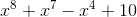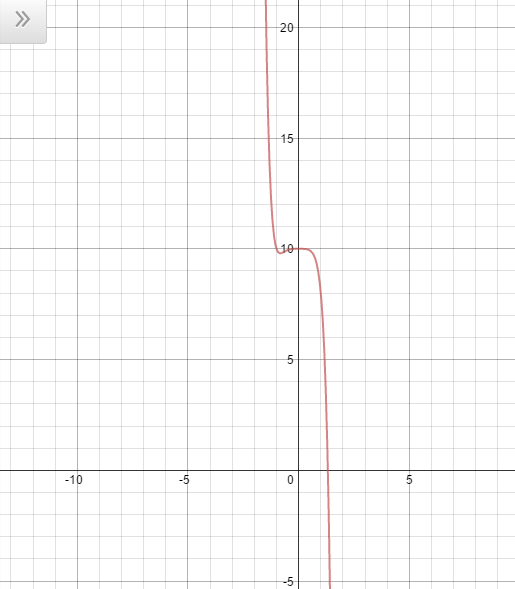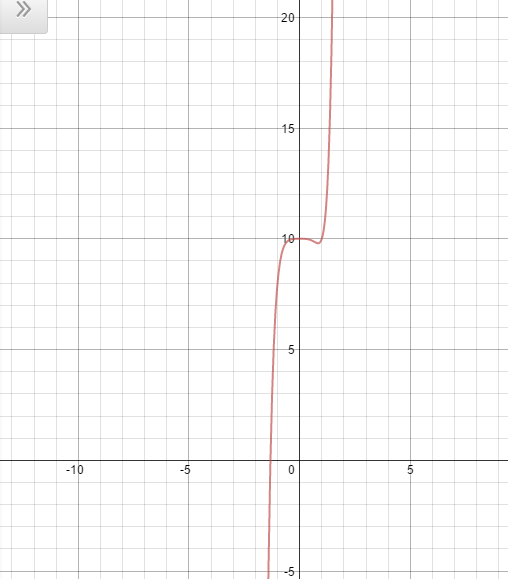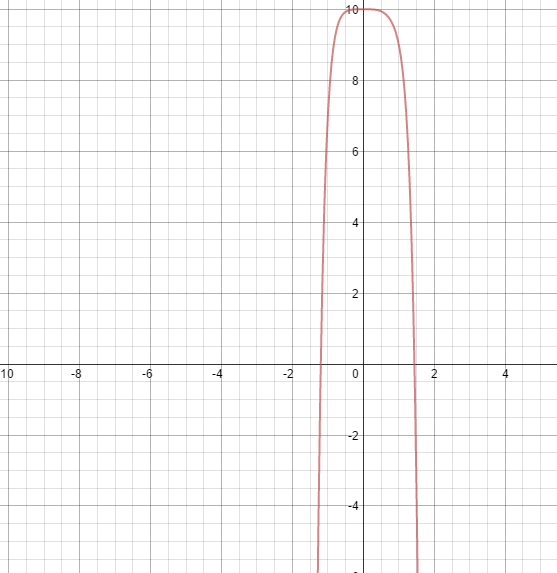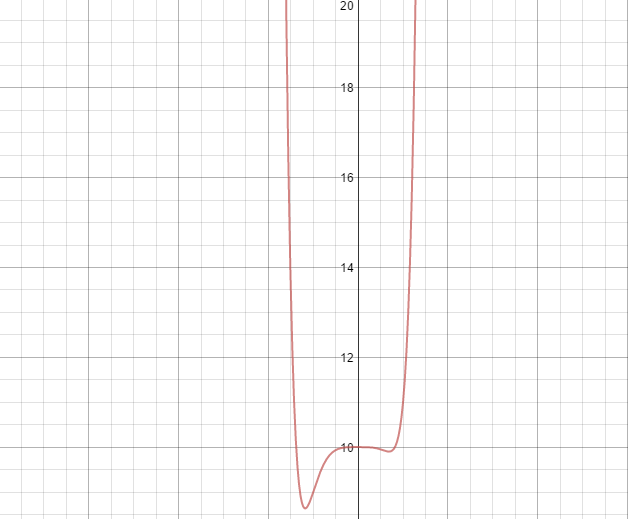Cannot be determinedExplanation:

The way to figure out this problem is by understanding behavior of polynomials.

The sign that occurs before the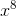is positive and therefore it is understood that the function will open upwards. the "8" on the function is an even number which means that the function is going to be u-shaped. The only answer choice that fits both these criteria is:### Example Question #141 : Functions And Graphs

Define a function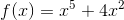.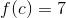for exactly one real value ofon the interval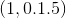.

Which of the following statements is correct about?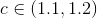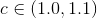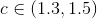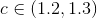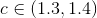Explanation:

Define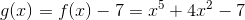. Then, if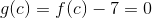, it follows that.

By the Intermediate Value Theorem (IVT), if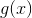is a continuous function, and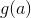and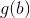are of unlike sign, then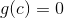for some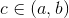. As a polynomial,is a continuous function, so the IVT applies here.

Evaluatefor each of the following values: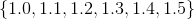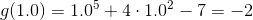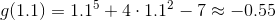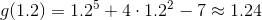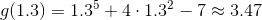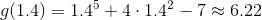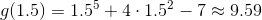Only in the case of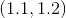does it hold thatassumes a different sign at both endpoints -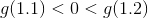. By the IVT,, and, for some.